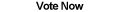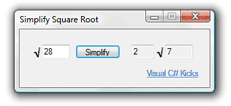Home Articles Components Code Snippets Links# C# Simplify Square Root## Simplify Square Roots

The C# programming language comes with a lot of math functions. They are basic however, and for more complex computations programmers need to write their own C# algorithms.

If you remember from math class, not all numbers are perfect squares. Meaning not all numbers are going to have a clean square root. For those numbers, it is possible to simplify square roots to get more accurate numbers than estimating.

## Cold Hard Math

First is the math. If you take the square root of 25, you get 5. Now take the square root of 28. There answer is about 5.29. That is an estimate and in some cases is nowhere good enough.

Unfortunately C# functions can only give that estimated answer. But now let's see how we can simplify the square root for a more accurate answer.

Let's instead look at 28 as the product of 4 * 7. The square root of 4 is 2. Thus the square root of 28 equals 2 * the square root of 7.

Now, can the square root of 5324 be simplified? This time let's use C# code to do it.

## C# Simplifying Square Roots

The C# algorithm basically looks for possible perfect-square numbers that divide evenly into your starting number. Of course you want to count backwards starting with the biggest number.

Here is a tip though, you only need to start with the integer value of the square root of the number. So if you set up an if loop, it would look something like this:

```for (int i = (int)Math.Floor(Math.Sqrt(inputNum)); i >= 2; i--)
{
}```

Then you would test each number to see if it's a multiple of the original number. If it is then you can safely take the square root of it and leave the remainder inside the square root sign.

The C# source code at the bottom has the full working algorithm to simplify square roots and a sample executable program...

Back to C# Article List